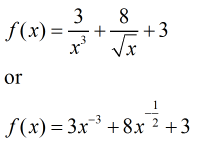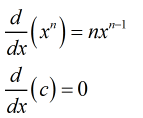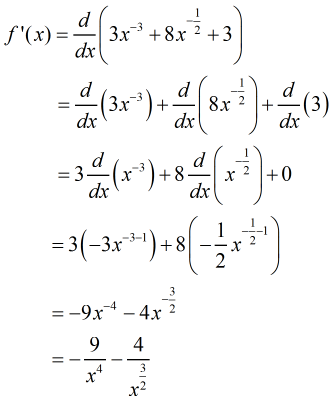# Find the derivative of the function by using the rules of differentiation.f(x) =  3/x3 + 8/√x + 3

Question
3 views
Find the derivative of the function by using the rules of differentiation.
f(x) =

3/x+ 8/√x + 3
check_circle

Step 1

Given function isStep 2

Using formulaStep 3

On differentiating f(x)...

### Want to see the full answer?

See Solution

#### Want to see this answer and more?

Solutions are written by subject experts who are available 24/7. Questions are typically answered within 1 hour.*

See Solution
*Response times may vary by subject and question.
Tagged in